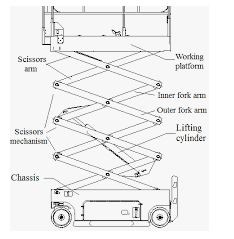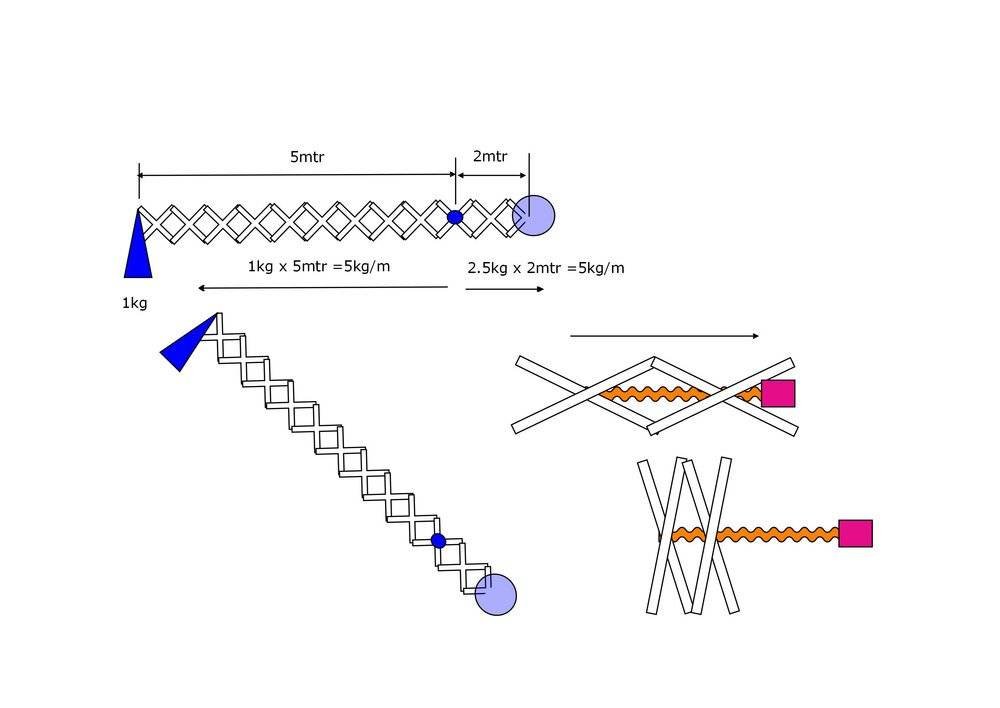# Fulcrum, balance point and scissor lift force

• cristofayre
In summary, the conversation discusses the basics of beams and fulcrums and how to calculate the counterweight needed for a scissor/accordion arrangement. The question also arises about the amount of force needed to push the pivots and the practicality of using a motor for this purpose. The individual asking the question is seeking a basic understanding of these concepts and formulas.

#### cristofayre

My first question, so be kind (!)

Long time since I was at school, and can only remember the basics of beams and fulcrums. In essence, that a 1kg weight 5mtrs from a fulcrum / pivot needs 5kg at 1mtr to counterbalance. But what about the beam itself? Do the two sides cancel each other out?

For example, I plan to create a "XXX" scissor / accordian arrangement. The metalwork is in ten "X" sections, each approx 1kg, and extends to 5mtrs. One kg weight on the end. The counterweight side has 1.5 "X" sections. All things equal, that gives a c/w of 3kg ... but doesn't account for the 10kg of beam metalwork. So how to calculate the counterweight required. Any ideas how to proceed?

Next (related) question. If the "X" were vertical, (they're not: At most 45 degrees) How much 'force' is needed to push the pivots in the middle of the "XX" apart. (My current thinking is a 8mm lead screw that has to rotate 40 times to cover the 300mm travel). If that's motorised, what sort of torque would that motor need? ... if it's even practical. Since the outer edges of the X are extending the next section, they must (in theory) be adding to the friction forces, (they will be bolts acting as pivots, not ball bearings)

Now whilst this question may appear that I have a smattering of knowledge on the subject, it's exactly that: basics. All the online formulaes that deal with "Newton/Meters", gravity, force, torque and all the other variables leave me out in the cold. So if poss, can you give any answer in "schoolboy" basics that someone of 12 ~ 14 could comprehend, (alas, I'm physically 4x that age range) Or simply say: You need XYZ for counterweight / ABC size for the motor

Hello @cristofayre ,!​

cristofayre said:
Do the two sides cancel each other out?
Nope !

cristofayre said:
The metalwork is in ten "X" sections, each approx 1kg, and extends to 5mtrs. One kg weight on the end. The counterweight side has 1.5 "X" sections.
Can you draw that for us ? I can't visualize a 'counterweight side' ?The first diagram is how the 'accordian' fits together, with 1kg at the front of 5mtrs, and the "1.5", (actually 2 sections) behind the pivot with a c/w. The blue circle is the fulcrum/pivot. Second picture is how it could operate, between this and horizontal, as well as extendding from 1mtr to 5mtr. Third pic is where I envisaged putting the motor to 'drive' the middle pivots to extend / decrease frame size.

Hope that helps

## 1. What is a fulcrum?

A fulcrum is a fixed point or pivot on which a lever or other mechanical device turns or is supported.

## 2. How does a fulcrum affect balance point?

The position of the fulcrum determines the balance point of a lever. Moving the fulcrum closer to the heavier end of the lever will result in a lower balance point, while moving it closer to the lighter end will result in a higher balance point.

## 3. What is a balance point?

A balance point, also known as the center of mass, is the point at which an object is in perfect balance. It is the point at which the weight of an object is evenly distributed on either side.

## 4. How does a scissor lift use force?

A scissor lift uses force by utilizing the principle of leverage. As the scissor arms extend, the force applied to the bottom of the arms increases, allowing the lift to raise heavy loads with relatively little force.

## 5. What is the relationship between the fulcrum and the force of a scissor lift?

The fulcrum of a scissor lift is the point where the lifting force, or effort, is applied. The closer the force is applied to the fulcrum, the more force is required to lift the load. This is why scissor lifts have a long, extended arm or lever to increase the distance between the force and the fulcrum, making it easier to lift heavy loads.